# Highest Common Factor of small integers

Let’s list all the factors of 12 and of 8:

Factors of 12 are: 1, 2, 3, 4, 6, 12

Factors of 8 are: 1, 2, 4, 8

By examining the lists, the highest factor common to both integers 4.

This kind of search is known as an exhaustive search – we list all the factors so all possible values are considered. For small numbers, this is a fast approach especially if it can be done mentally. For large numbers, it can be better to use the prime factor method.

# Lowest Common Multiple of small integers

Let’s find the lowest common multiple of 15 and 18.

Of course, the numberwhich is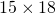is a common multiple of 15 and of 18. However, it is not the lowest common multiple.

Multiples of 15 are: 15, 30, 45, 60, 75, 90, 105, 120, …

Multiples of 18 are: 18, 36, 54, 72, 90, good, we can stop here.

The first multiple that we come to that is common to both is 90.

This method is good when the numbers are relatively small.

# Practice finding HCF and LCM of small integers

Practice finding the HCF and the LCM by recalling multiplication tables.

# Highest Common Factor using the prime factor method

Example 1: Let’s find the HCF of 24 and 30 using the prime factor method.Prime factors common to both 24 and 30 are 2 and 3. Thereforeis the HCF of 24 and 30.

Example 2: Let’s see how this works with much larger values:Common to both: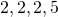That is, HCF of 360 and 400 is.

Notice that;. The numbersand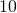are known as coprime – they don’t have any common factors.

# Lowest Common Multiple Formula

Find the LCM of 15 and 18.

Now,Note that:Therefore,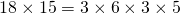Let’s divide both sides by 3, the highest common factor:We can be sure thatis a multiple of bothand(it isand). We can also be sure that it is the lowest common multiple as 5 and 6 do not share any factor other than 1.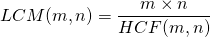Therefore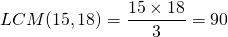# Practice finding HCF and LCM of larger integers

Use the prime factor method to find the HCF of the two numbers given. Then use the formula or otherwise to find the LCM.